• +91 9971497814
• info@interviewmaterial.com

# RD Chapter 18- Surface Areas and Volume of a Cuboid and Cube Ex-18.2 Interview Questions Answers

### Related Subjects

Question 1 : A cuboidal water tank is 6 m long, 5 m wide and 4.5 m deep. How many litres of water can it hold?

Length of water tank (l) = 6 m
and depth (h) = 4.5 m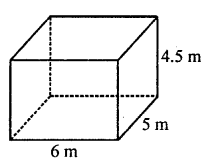∴ Volume of water in it = lbh
= 6 x 5 x 4.5 m3 = 135 m3
Capacity of water in litres = 135 x 1000 litres (1 m3 = 1000 l)
= 135000 litres

Question 2 : A cubical vessel is 10 m long and 8 m wide. How high must it be made to hold 380 cubic metres of a liquid? [NCERT]

Length of vessal (l) = 10 m
Volume = 380 m3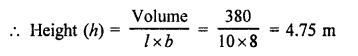Question 3 : Find the cost of digging a cuboidal pit 8 m long, 6 m broad and 3 m deep at the rate of ₹30 per m3.

Length of pit (l) = 8m
Width (b) = 6 m
and depth (h) = 3 m
∴ Volume of earth digout = lbh
= 8 x 6 x 3 = 144 m3
Cost of digging the pit at the rate of ₹30 per m3
= 144 x 30 = ₹4320

Question 4 : If the areas of three adjacent faces of a cuboid are 8 cm2, 18 cm2 and 25 cm2. Find the volume of the cuboid.

Let x, y, z be the three adjacent faces of the cuboid, then
x = 8 cm2, y = 18 cm2, z = 25 cm2
and let l, b, h are the dimensions of the cuboid, then
x = lb = 8 cm2
y = bh = 18 cm2
z = hl = 25 cm2
∴ Volume = lbh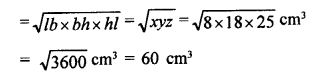Question 5 : The breadth of a room is twice its height, one half of its length and the volume of the room is 512 cu. dm. Find its dimensions.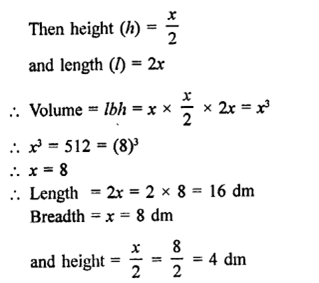Question 6 : Three metal cubes with edges 6 cm, 8 cm and 10 cm respectively are melted together and formed into a single cube. Find the volume, surface area and diagonal of the new cube.

Edge of first cube = 6 cm
Edge of second cube = 8 cm
and edge of third cube = 10 cm
∴ Volume of 3 cubes = (6)3 + (8)3 + (10)3 cm3
= 216 + 512 + 1000 cm3
= 1728 cm3
∴ Edge of so formed cube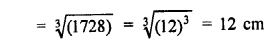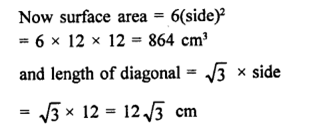Question 7 : Two cubes, each of volume 512 cm3 are joined end to end. Find the surface area of the resulting cuboid.

Volume of each volume = 512 cm3
∴ Side (edge) =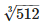=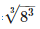= 8 cm
Now by joining two cubes, then Length of so formed cuboid (l)
= 8 + 8 = 16 cm
and height (h) = 8 cm
∴ Surface area = 2(lb + bh + hl)
= 2[16 x 8 + 8 x 8 + 8 x 16] cm2
= 2[128 + 64 + 128] cm2
= 2 x 320 = 640 cm2

Question 8 : A metal cube of edge 12 cm is melted and formed into three smaller cubes. If the edges of the two smaller cubes are 6 cm and 8 cm, find the edge of the third smaller cube.

Edge of metal cube = 12 cm
∴ Its volume = (Edge)3 = (12)3 cm33
= 1728 cm3
It is melted and form 3 cubes
Edge of one smaller cube = 6 cm
and edge of second smaller cube = 8 cm
∴ Volume of two smaller cubes = (6)3 + (8)3 cm3
= 216 + 512 cm3 = 728 cm3
∴ Volume of third smaller cube = 1728 – 728 = 1000 cm3
∴ Edge of the third cube =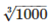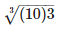cm = 10 cm

Question 9 : The dimensions of a cinema hall are 100 m, 50 m and 18 m. How many persons can sit in the hall, if each person requires 150 m3 of air?

Length of cinema hall (l) = 100 m
and height (h) = 18 m
∴ Volume of air in it = lbh
= 100 x 50 x 18 m3 = 90000 m3
Air required for one person = 150 m3
∴ Number of persons in the hall =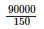= 600 persons

Question 10 : Given that 1 cubic cm of marble weighs 0.25 kg, the weight of marble block 28 cm in width and 5 cm thick is 112 kg. Find the length of the block.

Weight of 1 cm3 = 0.25 kg
Breadth of the block (b) = 28 cm
Thickness (h) = 5 cm
and total weight of the block = 112 kg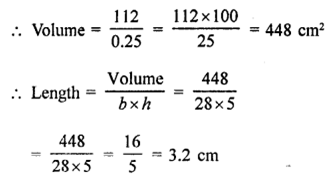Todays Deals### RD Chapter 18- Surface Areas and Volume of a Cuboid and Cube Ex-18.2 Contributorskrishan

Name:
Email:

# Latest News# 9000 interview questions in different categories